# JP5327656B2 - Physical quantity detection device and inspection method for physical quantity detection device - Google Patents

Physical quantity detection device and inspection method for physical quantity detection device Download PDF

## Info

Publication number
JP5327656B2
JP5327656B2 JP2011154657A JP2011154657A JP5327656B2 JP 5327656 B2 JP5327656 B2 JP 5327656B2 JP 2011154657 A JP2011154657 A JP 2011154657A JP 2011154657 A JP2011154657 A JP 2011154657A JP 5327656 B2 JP5327656 B2 JP 5327656B2
Authority
JP
Japan
Prior art keywords
value
error amount
physical quantity
actual output
correction value
Prior art date
Legal status (The legal status is an assumption and is not a legal conclusion. Google has not performed a legal analysis and makes no representation as to the accuracy of the status listed.)
Active
Application number
JP2011154657A
Other languages
Japanese (ja)
Other versions
JP2013019829A (en
Inventor

Original Assignee

Priority date (The priority date is an assumption and is not a legal conclusion. Google has not performed a legal analysis and makes no representation as to the accuracy of the date listed.)
Filing date
Publication date
Application filed by 株式会社デンソー filed Critical 株式会社デンソー
Priority to JP2011154657A priority Critical patent/JP5327656B2/en
Publication of JP2013019829A publication Critical patent/JP2013019829A/en
Application granted granted Critical
Publication of JP5327656B2 publication Critical patent/JP5327656B2/en
Application status is Active legal-status Critical
Anticipated expiration legal-status Critical

• 238000004364 calculation methods Methods 0 abstract 3
• 230000000875 corresponding Effects 0 abstract 1
• 238000000034 methods Methods 0 abstract 1

## Images

••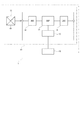••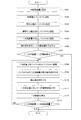•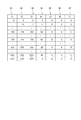•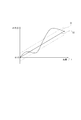•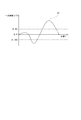•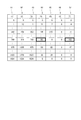•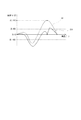••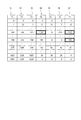•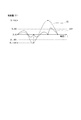•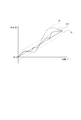•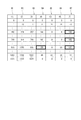•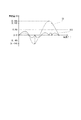•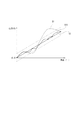•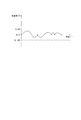## Classifications

• GPHYSICS
• G01MEASURING; TESTING
• G01DMEASURING NOT SPECIALLY ADAPTED FOR A SPECIFIC VARIABLE; ARRANGEMENTS FOR MEASURING TWO OR MORE VARIABLES NOT COVERED IN A SINGLE OTHER SUBCLASS; TARIFF METERING APPARATUS; MEASURING OR TESTING NOT OTHERWISE PROVIDED FOR
• G01D5/00Mechanical means for transferring the output of a sensing member; Means for converting the output of a sensing member to another variable where the form or nature of the sensing member does not constrain the means for converting; Transducers not specially adapted for a specific variable
• G01D5/12Mechanical means for transferring the output of a sensing member; Means for converting the output of a sensing member to another variable where the form or nature of the sensing member does not constrain the means for converting; Transducers not specially adapted for a specific variable using electric or magnetic means
• G01D5/14Mechanical means for transferring the output of a sensing member; Means for converting the output of a sensing member to another variable where the form or nature of the sensing member does not constrain the means for converting; Transducers not specially adapted for a specific variable using electric or magnetic means influencing the magnitude of a current or voltage
• G01D5/142Mechanical means for transferring the output of a sensing member; Means for converting the output of a sensing member to another variable where the form or nature of the sensing member does not constrain the means for converting; Transducers not specially adapted for a specific variable using electric or magnetic means influencing the magnitude of a current or voltage using Hall-effect devices
• G01D5/145Mechanical means for transferring the output of a sensing member; Means for converting the output of a sensing member to another variable where the form or nature of the sensing member does not constrain the means for converting; Transducers not specially adapted for a specific variable using electric or magnetic means influencing the magnitude of a current or voltage using Hall-effect devices influenced by the relative movement between the Hall device and magnetic fields
• GPHYSICS
• G01MEASURING; TESTING
• G01BMEASURING LENGTH, THICKNESS OR SIMILAR LINEAR DIMENSIONS; MEASURING ANGLES; MEASURING AREAS; MEASURING IRREGULARITIES OF SURFACES OR CONTOURS
• G01B21/00Measuring arrangements or details thereof in so far as they are not adapted to particular types of measuring means of the preceding groups
• GPHYSICS
• G01MEASURING; TESTING
• G01BMEASURING LENGTH, THICKNESS OR SIMILAR LINEAR DIMENSIONS; MEASURING ANGLES; MEASURING AREAS; MEASURING IRREGULARITIES OF SURFACES OR CONTOURS
• G01B21/00Measuring arrangements or details thereof in so far as they are not adapted to particular types of measuring means of the preceding groups
• G01B21/22Measuring arrangements or details thereof in so far as they are not adapted to particular types of measuring means of the preceding groups for measuring angles or tapers; for testing the alignment of axes
• GPHYSICS
• G01MEASURING; TESTING
• G01DMEASURING NOT SPECIALLY ADAPTED FOR A SPECIFIC VARIABLE; ARRANGEMENTS FOR MEASURING TWO OR MORE VARIABLES NOT COVERED IN A SINGLE OTHER SUBCLASS; TARIFF METERING APPARATUS; MEASURING OR TESTING NOT OTHERWISE PROVIDED FOR
• G01D18/00Testing or calibrating of apparatus or arrangements provided for in groups G01D1/00 - G01D15/00
• G01D18/008Testing or calibrating of apparatus or arrangements provided for in groups G01D1/00 - G01D15/00 with calibration coefficients stored in memory

## Abstract

A physical quantity detection apparatus includes a signal output section, a correction value calculation section, and a processing section. The signal output section outputs signals in accordance with a change in physical quantity of a detected object. The correction value calculation section calculates a correction value. The processing section corrects values based on actual output values of the signal output section with the correction value, calculates physical quantities of the detected object based on corrected values, and outputs calculated physical quantities. The correction value calculation section calculates the correction value based on primary error amounts that are differences between post-interpolation actual output values calculated by executing a linear function interpolation process to the values based on the actual output values within a predetermined physical quantity range and the values based on the actual output values corresponding to the post-interpolation actual output values.

## Description

The present invention relates to a physical quantity detection device and an inspection method for a physical quantity detection device.

Conventionally, by installing one of a magnetic generation means such as a magnet and a magnetic flux density detection means such as a Hall element as a detection target, and detecting the magnetism of the magnetic generation means when the detection target rotates, the magnetic flux density detection means A rotation angle detection device that detects a rotation angle of a detection target is known.
For example, the rotation angle detection device disclosed in Patent Document 1 includes a magnet as a magnetism generation unit, a magnetic flux density sensor as a magnetic flux density detection unit, and a signal processing unit as a processing unit, and the signal processing unit performs correction calculation. Do. The signal processing unit corrects the actual output voltage based on the output signal of the magnetic flux density sensor based on a predetermined value (voltage level) corresponding to a preset correction point. Here, the various correction points set in advance are set at equal intervals.

JP 2002-206911 A JP 2007-71889 A Japanese Patent No. 3491777

By the way, when the interval between the correction points is constant, the nonlinearity of the output signal is not improved, and the error of the actual output voltage remains as the linearity error of the rotation angle. Here, Patent Document 2 describes a correction method that finely divides a portion where the output change rate is larger than a portion where the output change rate is small, and Patent Document 3 converts a digital signal to the output signal of the magnetic detection element. A correction method is described in which conversion is performed and arithmetic processing is performed with an inverse sine function. However, when the output waveform is unknown, the correction methods described in Patent Document 2 and Patent Document 3 cannot be applied.
The present invention has been made in view of the above-described problems, and provides a physical quantity detection device capable of accurately correcting an actual output value without being affected by the shape of an output waveform, and an inspection method for the physical quantity detection device. There is to do.

According to the first aspect of the present invention, the physical quantity detection device includes a signal output unit, a processing unit, and a predetermined correction value calculation unit. The signal output means outputs a signal corresponding to a change in the physical quantity of the detected object. The predetermined correction value calculating means calculates a predetermined correction value. The processing unit corrects a value based on an actual output value by the signal output unit based on a predetermined correction value stored in advance, calculates a physical quantity of the detection target based on the corrected value, and outputs the calculated physical quantity. The predetermined correction value calculation means corresponds to the post-interpolation actual output value calculated by performing the linear function interpolation process on the value based on the actual output value within the predetermined physical quantity range, and the post-interpolation actual output value. A predetermined correction value is set based on a primary error amount that is a difference from a value based on the actual output value.
Thus, by setting a predetermined correction value based on the primary error amount, an optimal correction point can be obtained, and the actual output value can be accurately corrected without being affected by the shape of the output waveform. . Further, since the predetermined correction value is obtained by performing the linear function interpolation process on the actual output value, the calculation can be simplified and the processing time can be shortened.

The value based on the actual output value is a value obtained by processing the actual output value with an inverse sine function or an inverse cosine function.
Thereby, the linearity error of the actual output value can be further reduced.

Further , the predetermined correction value calculation means is a secondary that is a difference between the post-interpolation error amount calculated by performing the linear function interpolation process on the primary error amount and the primary error amount corresponding to the post-interpolation error amount. A maximum absolute value calculation process for calculating the maximum absolute value of the error amount is performed, and the primary error amount corresponding to the maximum absolute value of the secondary error amount is set as a predetermined correction value.
Thereby, since the primary error amount corresponding to the maximum absolute value of the secondary error amount is always set as the predetermined correction value, it is possible to set the predetermined correction value and the correction point in order from the portion with the larger error amount. Therefore, the optimal predetermined correction value and correction point can be set.

According to the second aspect of the present invention, the predetermined correction value calculating means performs the maximum absolute value calculation process and the primary error amount corresponding to the maximum absolute value of the secondary error amount until the secondary error amount becomes smaller than the predetermined threshold value. Is repeatedly set as a predetermined correction value .
Thereby, it can suppress that processing time becomes long because the maximum absolute value calculation processing frequency increases.

According to the invention of claim 3 , the physical quantity is a relative rotation angle or a relative stroke quantity.
Thereby, the actual output value of the relative rotation angle or the relative stroke amount can be accurately corrected without being affected by the shape of the output waveform.

According to the invention of claim 4, a method of inspecting a physical quantity detecting device according to any one of claims 1 to 3, the physical quantity when the processing number of the maximum absolute value calculation process is equal to or greater than a predetermined number of times It is determined that the detection device is defective.
Thereby, a defective physical quantity detection device can be easily found.

The schematic diagram which shows the rotation angle detection apparatus of one Embodiment of this invention. The schematic diagram which shows the processing circuit of the rotation angle detection apparatus of one Embodiment of this invention. The figure which shows the predetermined | prescribed correction value of the rotation angle detection apparatus of one Embodiment of this invention. The flowchart which calculates the predetermined | prescribed correction value of one Embodiment of this invention. The figure which shows the content of the buffer for demonstrating the process which calculates the predetermined | prescribed correction value of one Embodiment of this invention. The characteristic view which shows the relationship between the angle of the rotation angle detection apparatus of one Embodiment of this invention, and an output. The characteristic view which shows the relationship between the angle of the rotation angle detection apparatus of one Embodiment of this invention, and an error amount. The figure which shows the content of the buffer for demonstrating the process which calculates the predetermined | prescribed correction value of one Embodiment of this invention. The characteristic view which shows the relationship between the angle of the rotation angle detection apparatus of one Embodiment of this invention, and an error amount. The characteristic view which shows the relationship between the angle of the rotation angle detection apparatus of one Embodiment of this invention, and an output. The figure which shows the content of the buffer for demonstrating the process which calculates the predetermined | prescribed correction value of one Embodiment of this invention. The characteristic view which shows the relationship between the angle of the rotation angle detection apparatus of one Embodiment of this invention, and an error amount. The characteristic view which shows the relationship between the angle of the rotation angle detection apparatus of one Embodiment of this invention, and an output. The figure which shows the content of the buffer for demonstrating the process which calculates the predetermined | prescribed correction value of one Embodiment of this invention. The characteristic view which shows the relationship between the angle of the rotation angle detection apparatus of one Embodiment of this invention, and an error amount. The characteristic view which shows the relationship between the angle of the rotation angle detection apparatus of one Embodiment of this invention, and an output. The characteristic view which shows the relationship between the angle of the rotation angle detection apparatus of one Embodiment of this invention, and an error amount.

Hereinafter, physical quantity detection devices according to a plurality of embodiments of the present invention will be described with reference to the drawings.
(First embodiment)
In the first embodiment of the present invention, a physical quantity detection device is applied to a rotation angle detection device. A rotation angle detection device according to a first embodiment of the present invention is shown in FIG. The rotation angle detection device 1 is a device that detects a relative rotation angle of, for example, a throttle valve shaft as a detection target. Here, the relative rotation angle corresponds to “physical quantity” in the claims. The rotation angle detection device 1 includes a permanent magnet 20, a Hall IC 10 including a Hall element 11, a digital signal processor (hereinafter referred to as DSP) 12, and a memory 13.

The permanent magnet 20 is attached to a yoke 30 as a detected body provided on the valve shaft of the throttle. The permanent magnet 20 is provided to be rotatable relative to the Hall IC 10 as the yoke 30 rotates.
The Hall element 11 is formed of a semiconductor thin film and corresponds to “signal output means” in the claims. The Hall element 11 outputs a signal corresponding to a change in magnetic flux density.

The DSP 12 specializes in digital signal processing, and performs processing such as correction processing and rotation angle calculation processing on the value output from the Hall element 11 and converted into a digital signal. The DSP 12 corresponds to a “processing unit” in the claims.

The memory 13 includes, for example, a read-only memory and a writable / erasable memory, and stores various data used by the DSP 12. The memory 13 stores predetermined correction values K (1) to K (m) corresponding to the rotation angle of the yoke 30 (see FIG. 3). Here, m is a natural number greater than 1.

In the present embodiment, as shown in FIG. 2, the Hall IC 10 includes, in addition to the Hall element 11, the DSP 12, and the memory 13, an analog-digital conversion circuit (hereinafter referred to as ADC) 14, and a digital-analog conversion circuit. (Hereinafter referred to as DAC) 15 or the like. The Hall IC 10 is provided such that the magnetosensitive surface of the Hall element 11 is positioned on the central axis O (see FIG. 1).

The computer 16 is provided outside the Hall IC 10, calculates predetermined correction values K (1) to K (m), and stores them in the memory 13. The computer 16 corresponds to “predetermined correction value calculating means” in the claims.

Next, the operation of the rotation angle detection device 1 will be described.
The Hall element 11 outputs a signal corresponding to a change in magnetic flux density caused by relative rotation around the central axis O with respect to the permanent magnet 20. The ADC 14 converts an analog value output from the Hall element 11 into a digital value and transmits the digital value to the DSP 12. Hereinafter, the digital value converted by the ADC 14 is simply referred to as an actual output value. The DSP 12 performs correction processing, rotation angle calculation processing, and the like on the output value from the Hall element 11. Further, the DSP 12 transmits the processing result to the DAC 15. The DAC 15 converts the digital value transmitted from the DSP 12 into an analog value and outputs the analog value.
A correction process by the DSP 12 will be described.
In the present embodiment, for example, m correction points are set in advance, and the actual output value is corrected based on a predetermined value corresponding to the m correction points. The memory 13 stores predetermined values A (1) to A (m) and predetermined correction values K (1) to K (m) corresponding to the respective correction points. As shown in FIG. 3, the predetermined values A (1) to A (m) and the predetermined correction values K (1) to K (m) are associated with each other. The predetermined values A (1) to A (m) are all values within the range of the actual output value (voltage level) of the Hall element 11. Here, the “predetermined physical quantity range” in the claims corresponds to an angular range in which the detection target can be rotated.

The DSP 12 corrects the actual output value of the Hall element 11 based on the predetermined values A (1) to A (m) and the predetermined correction values K (1) to K (m).
When the actual output value matches any one of the predetermined values A (1) to A (m), a predetermined correction value corresponding to the predetermined value matching the actual output value is subtracted from the actual output value. Correct the actual output value. For example, when the actual output value matches A (3), the predetermined correction value corresponding to A (3) is K (3) as shown in FIG. It is corrected as K (3).

When the actual output value is different from any of the predetermined values A (1) to A (m), the actual output value is corrected by subtracting the operation correction value corresponding to the actual output value from the actual output value. The calculation correction value K is linearly interpolated by the following equation 1 derived from the following equation 1 using two predetermined values between the actual output values and the predetermined correction values corresponding to the two predetermined values. Is calculated by
{K (n) -K (n-1)} / {A (n) -A (n-1)} = {KK (n-1)} / {A-A (n-1)} ..Formula 1
K = {K (n) -K (n-1)} / {A (n) -A (n-1)} * {AA (n-1)} + K (n-1) Expression 2

For example, when the actual output value is a value A between the predetermined value A (3) and the predetermined value A (4), the calculation correction value corresponding to the actual output value A is K. Here, when the actual output value A, the predetermined value A (3), the predetermined value A (4), the predetermined correction value K (3), and the predetermined correction value K (4) are substituted into Expression 1, Expression 3 is obtained. .
{K (4) -K (3)} / {A (4) -A (3)} = {KK (3)} / {AA (3)} Expression 3
The following formula 4 is obtained by the formula 3.
K = [{K (4) −K (3)} / {A (4) −A (3)}] × {AA− (3)} + K (3) Equation 4
Further, since the actual output value is corrected to AK, the actual output value is corrected to a calculated value according to the following equation 5.
A-[{K (4) -K (3)} / {A (4) -A (3)}] × {AA (3)}-K (3) Formula 5
In this way, the DSP 12 corrects the actual output value by subtracting the calculation correction value calculated by the linear function interpolation process from the actual output value, and calculates the rotation angle.

Hereinafter, the setting of the predetermined correction value will be described in detail with reference to FIGS.
In the present embodiment, the correction value is calculated using the computer 16 provided outside the Hall IC 10. The computer 16 calculates a predetermined correction value based on the processing flowchart shown in FIG.
In S101, a target error amount is set. In the present embodiment, for example, the target error amount is set to 80.

In S102, the angle value corresponding to the angle within the rotatable angle range of the yoke 30 is stored in the buffer B1. In the present embodiment, the rotatable angle range of the yoke 30 is 90 degrees. Further, the angle value 0 stored in the buffer B1 shown in FIG. 5 corresponds to 0 degree, and the angle value 1024 corresponds to 90 degrees. That is, one scale of the value stored in the buffer B1 corresponds to 90/1024 degrees.

In S103, the actual output value output from the Hall element 11 is detected, and the actual output value corresponding to the rotation angle of the yoke 30 is stored in the buffer B2. As shown in FIG. 6, the actual output value of the present embodiment is represented by a curve S1 passing through the point (0, 0).

In S104, an ideal output value is calculated based on the actual output value detected by the Hall element 11. In the present embodiment, the ideal output value is a value on a straight line represented by a linear function connecting the maximum value and the minimum value of the actual output value. As shown in FIG. 6, the ideal output value of this embodiment is represented by a straight line S2 passing through the point (0, 0). The calculated ideal output value is stored in the buffer B3. Here, the ideal output value corresponds to “actual output value after interpolation” in the claims.

In S105, the primary error amount of the actual output value is calculated. The primary error amount of the actual output value is the difference between the actual output value and the ideal output value. Here, the difference between the actual output value stored in the buffer B2 and the ideal output value stored in the buffer B3 is calculated and stored in the buffer B4. As shown in FIG. 7, a primary error amount corresponding to an angle within a predetermined angle range is represented by a curve S3.

In S106, a correction value is set, and a linear function interpolation process is performed on the primary error amount. Here, the maximum absolute value of the primary error amount stored in the buffer B4 is set as the correction value and stored in the corresponding area of the buffer B7. As shown in FIGS. 5 and 8, the maximum absolute value of the primary error amount stored in the buffer B4 is 165. Therefore, in this process, 165 stored in the buffer B4 is set as a correction value and stored in the corresponding area of the buffer B7. In S106, linear function interpolation processing is performed on the primary error amount between 165 and 0 in the buffer B4, and the calculated value is set as the post-interpolation error amount and stored in the corresponding area of the buffer B7. Subsequently, the difference between the value stored in the buffer B4 and the value stored in the corresponding area of the buffer B7 is set as a secondary error amount and stored in the buffer B5. Here, as shown in FIG. 9, the secondary error amount stored in the buffer B5 is represented by a curve S31. Further, as shown in FIG. 10, the actual output value corrected by the correction value 165 is represented by a curve S11.

In S107, it is determined whether or not the absolute values of all secondary error amounts stored in the buffer B5 are smaller than a predetermined value. If the secondary error amount is smaller than the predetermined value (S107: YES), the process ends. On the other hand, when the secondary error amount is larger than the predetermined value (S107: NO), the process proceeds to S108. After the processing, as shown in FIGS. 8 and 9, since there is a secondary error amount whose absolute value is larger than the target error amount 80 in the buffer B5, the processing moves to S108.

In S108, the secondary error amount stored in the buffer B5 is copied to the buffer B6. After the processing, as shown in FIGS. 8 and 11, the secondary error amount stored in the buffer B5 is duplicated in the buffer B6.

In S109, the maximum absolute value of the secondary error amount stored in the buffer B6 is calculated. Here, as shown in FIG. 11, the maximum absolute value of the secondary error amount stored in the buffer B6 is 215.

In S110, a correction value is set. Here, the primary error amount corresponding to the maximum absolute value of the secondary error amount detected in S109 is set as a correction value and stored in the corresponding area of the buffer B7. After the processing, as shown in FIG. 11, -144 of the buffer B4 corresponding to -215 of the buffer B6 is stored in the corresponding region of the buffer B7.

In S111, a linear function interpolation process is performed on the primary error amount. Here, linear function interpolation processing is performed on the primary error amount corresponding to the secondary error amount between the maximum absolute value in the buffer B6 and the maximum absolute value in the buffer B6 and the nearest zero value, and is calculated. The stored value is stored in the corresponding area of the buffer B7. In the present embodiment, as shown in FIG. 11, a linear function with respect to the primary error amount corresponding to the angle value 0 to the angle value 302 and the primary error amount corresponding to the angle value 302 to the angle value 749 in the buffer B4. Interpolation processing is performed, and the calculated value is set as the post-interpolation error amount and stored in the corresponding area of the buffer B7.

In S112, a secondary error amount is calculated. Here, the difference between the primary error amount stored in the buffer B4 and the post-interpolation error amount stored in the corresponding buffer B7 is set as a new secondary error amount and stored in the buffer B5. After the processing, as shown in FIG. 11, the value in the buffer B5 is rewritten by the difference between the value in the buffer B4 and the value in the buffer B7. Here, as shown in FIG. 12, the secondary error amount stored in the buffer B <b> 5 is represented by a curve 32. Further, as shown in FIG. 13, the actual output value corrected by the correction values 165 and -144 is represented by a curve S12.

In S113, it is determined whether the absolute value of the secondary error amount in the entire area is smaller than the target error amount. If the absolute value of the secondary error amount in the entire area is smaller than the target error amount (S113: YES), the process ends. On the other hand, when the absolute value of the secondary error amount in the entire area is not smaller than the target error amount (S113: NO), the process proceeds to S108. After the process, as shown in FIGS. 11 and 12, since the absolute value remains larger than the target error amount 80 in the buffer B5, the process proceeds to S108.

In S108, the secondary error amount stored in the buffer B5 is copied to the buffer B6. After the processing, as shown in FIGS. 11 and 14, the secondary error amount stored in the buffer B5 is duplicated in the buffer B6.

In S109, the maximum absolute value of the secondary error amount stored in the buffer B6 is detected. As shown in FIG. 14, the maximum absolute value of the secondary error amount stored in the buffer B6 is 83.

In S110, a correction value is set. Here, the primary error amount corresponding to the maximum absolute value of the secondary error amount detected in S109 is set as a correction value and stored in the corresponding area of the buffer B7. After the processing, as shown in FIG. 14, the primary error amount 130 of the buffer B4 corresponding to the maximum absolute value 83 of the buffer B6 is stored in the corresponding area of the buffer B7.

In S111, a linear function interpolation process is performed on the primary error amount. Here, linear function interpolation processing is performed on the primary error amount corresponding to the secondary error amount between the maximum absolute value in the buffer B6 and the maximum absolute value in the buffer B6 and the nearest zero value, and is calculated. The stored value is stored in the corresponding area of the buffer B7. As shown in FIG. 14, the angle value corresponding to the maximum absolute value 83 in the buffer B6 is 875, and the angle values corresponding to the 0 value closest to the maximum absolute value 83 in the buffer B6 are 749 and 1024. Here, a linear function interpolation process is performed on the primary error amount corresponding to the angle value 749 to the angle value 875 and the primary error amount corresponding to the angle value 875 to the angle value 1024 in the buffer B4, and the calculated value Are stored in the corresponding area of the buffer B7.

In S112, a new secondary error amount is calculated. After the processing, as shown in FIG. 14, the value in the buffer B5 is rewritten to the difference between the value in the buffer B4 and the value in the buffer B7. Here, as shown in FIG. 15, the secondary error amount stored in the buffer B5 is represented by S33. Further, as shown in FIG. 16, the actual output value corrected by the correction values 165, −144, 83 is represented by a curve 33.

In S113, it is determined whether the absolute value of the secondary error amount in the entire area is smaller than the target error amount 80. After the determination, as shown in FIG. 14, since the secondary error amount whose absolute value is larger than the target error amount 80 does not remain in the buffer B5, the process ends.

Here, the processing from S108 to S113 is repeated until the absolute value of the secondary error amount becomes smaller than the target error amount 80. The processing from S108 to S113 corresponds to “maximum absolute value calculation processing” in the claims.

Next, an inspection method of the rotation angle detection device 1 will be described.
In the present embodiment, it is determined that the rotation angle detection device 1 is defective when the maximum absolute value calculation processing count becomes a predetermined number or more until the absolute value of the secondary error amount becomes smaller than the target error amount 80. Here, for example, when the maximum absolute value calculation processing count is 6 or more, it is determined that the rotation angle detection device 1 is defective.

In the present embodiment, the primary error amount corresponding to the maximum absolute value of the secondary error amount of the actual output value is set as the correction value. As a result, an optimum correction point can be obtained, and the actual output value can be accurately corrected without being affected by the shape of the output waveform. FIG. 17 shows the error amount of the actual output value corrected by the correction values 165, -144, 83. As shown in FIG. 17, in the present embodiment, correction with high accuracy can be performed on the actual output value with a few correction points.
Further, since the primary error amount corresponding to the maximum absolute value of the secondary error amount is always set as the predetermined correction value, it is possible to set the predetermined correction value and the correction point in order from the location where the error amount is large. Therefore, the optimal predetermined correction value and correction point can be set.
Furthermore, since the predetermined correction value is obtained by performing the linear function interpolation process on the actual output value, the calculation can be simplified and the processing time can be shortened.

In the present embodiment, a target error amount 80 is set. As a result, an increase in the maximum absolute value calculation processing count prevents an increase in processing time.

In this embodiment, when the predetermined correction value is calculated until it becomes smaller than the target error amount 80, if the number of the predetermined correction values is equal to or larger than the predetermined number, it is determined that the rotation angle detection device 1 is defective. Thereby, the defective rotation angle detection apparatus 1 can be found easily.

(Other embodiments)
In the above embodiment, the physical quantity detection device is applied to the rotation angle detection device. On the other hand, in another embodiment, the physical quantity detection device may be applied to the stroke amount detection device. Further, the present invention may be applied to a physical quantity detection device that corrects another actual curve linear output value.
In the above embodiment, the computer as the predetermined correction value calculation means for calculating the predetermined correction value is provided outside the Hall IC. On the other hand, in another embodiment, an arithmetic unit such as a microcomputer as a predetermined correction value calculation unit may be provided in the Hall IC.
In the above embodiment, the predetermined correction value calculating means sets the predetermined correction value by performing the maximum absolute value calculation process on the primary error amount of the actual output value, and the processing unit sets the predetermined correction value on the actual output value. To perform correction processing. On the other hand, in another embodiment, the predetermined correction value calculating means performs the maximum absolute value calculation process on the primary error amount obtained by processing the actual output value with the inverse sine function or the inverse cosine function, thereby determining the predetermined correction value. And the correction processing may be performed using a predetermined correction value for the value obtained by processing the actual output value with the inverse sine function or the inverse cosine function.
The present invention described above is not limited to the above-described embodiment, and can be applied to various embodiments without departing from the gist thereof.

1 ・ ・ ・ Rotation angle detector (physical quantity detector)
11 ... Hall element (signal output means)
12 ... DSP (Processor)
16: Computer (predetermined correction value calculation means)
30 ... Yoke (object to be detected)

## Claims (4)

1. Signal output means for outputting a signal corresponding to a change in the physical quantity of the detected object;
A predetermined correction value calculating means for calculating the predetermined correction value;
A processing unit that corrects a value based on an actual output value by the signal output unit based on the predetermined correction value stored in advance, and calculates and outputs a physical quantity of the detected object based on the corrected value;
With
The predetermined correction value calculating means includes
And the actual output value after interpolation is calculated by performing a linear interpolation process on value based on the actual output values in Jo Tokoro physical quantity range, the value based on the actual output value corresponding after the interpolation actual output value set the predetermined correction value based on the primary error amount which is a difference between,
The maximum absolute value of the secondary error amount, which is the difference between the post-interpolation error amount calculated by performing a linear function interpolation process on the primary error amount and the primary error amount corresponding to the post-interpolation error amount, Perform a maximum absolute value calculation process to calculate, the primary error amount corresponding to the maximum absolute value of the secondary error amount as the predetermined correction value,
The physical quantity detection device according to claim 1, wherein the value based on the actual output value is a value obtained by processing the actual output value with an inverse sine function or an inverse cosine function .
2. The predetermined correction value calculating means includes
Until the secondary error amount becomes smaller than a predetermined threshold value, the maximum absolute value calculation process and the primary error amount corresponding to the maximum absolute value of the secondary error amount are repeatedly set as a predetermined correction value. The physical quantity detection device according to claim 1 .
3. The physical quantity is a physical quantity detecting device according to claim 1 or 2, wherein the relative rotational angle, or a relative stroke amount.
4. An inspection method for a physical quantity detection device according to any one of claims 1 to 3 ,
An inspection method for a physical quantity detection device, wherein the physical quantity detection device determines that the physical quantity detection device is defective when the maximum absolute value calculation processing is performed a predetermined number of times or more.
JP2011154657A 2011-07-13 2011-07-13 Physical quantity detection device and inspection method for physical quantity detection device Active JP5327656B2 (en)

## Priority Applications (1)

Application Number Priority Date Filing Date Title
JP2011154657A JP5327656B2 (en) 2011-07-13 2011-07-13 Physical quantity detection device and inspection method for physical quantity detection device

## Applications Claiming Priority (4)

Application Number Priority Date Filing Date Title
JP2011154657A JP5327656B2 (en) 2011-07-13 2011-07-13 Physical quantity detection device and inspection method for physical quantity detection device
US13/546,068 US9127966B2 (en) 2011-07-13 2012-07-11 Physical quantity detection apparatus and inspection method of physical quantity detection apparatus
DE201210212226 DE102012212226A1 (en) 2011-07-13 2012-07-12 A physical quantity detection device and a physical quantity detection device inspection method
CN201210243409.7A CN102878921B (en) 2011-07-13 2012-07-13 The inspection method of physical quantity detection device and physical quantity detection device

## Publications (2)

Publication Number Publication Date
JP2013019829A JP2013019829A (en) 2013-01-31
JP5327656B2 true JP5327656B2 (en) 2013-10-30

# Family

## Family Applications (1)

Application Number Title Priority Date Filing Date
JP2011154657A Active JP5327656B2 (en) 2011-07-13 2011-07-13 Physical quantity detection device and inspection method for physical quantity detection device

## Country Status (4)

US (1) US9127966B2 (en)
JP (1) JP5327656B2 (en)
CN (1) CN102878921B (en)
DE (1) DE102012212226A1 (en)

## Families Citing this family (5)

* Cited by examiner, † Cited by third party
Publication number Priority date Publication date Assignee Title
JP5839238B2 (en) * 2013-04-11 2016-01-06 株式会社デンソー Rotation angle detector
JP2014222162A (en) * 2013-05-13 2014-11-27 株式会社デンソー Sensor circuit and sensor
SI24774A (en) * 2014-07-11 2016-01-29 RLS merilna tehnika d.o.o. Poslovna cona Ĺ˝eje pri Komendi Process and device for adjusting rotary encoder
JP6217596B2 (en) * 2014-11-04 2017-10-25 株式会社デンソー Rotation angle and stroke amount detection device
JP2019078547A (en) * 2017-10-20 2019-05-23 株式会社デンソー Position detector

## Family Cites Families (18)

* Cited by examiner, † Cited by third party
Publication number Priority date Publication date Assignee Title
US5946356A (en) * 1997-07-16 1999-08-31 Motorola, Inc. Method and apparatus for data transmission within a broad-band communications system
US6370480B1 (en) * 1999-05-21 2002-04-09 General Electric Company Quantitative analysis system and method for certifying ultrasound medical imaging equipment
US6401052B1 (en) * 1999-08-02 2002-06-04 Siemens Aktiengesellschaft Determination method for a position signal and/or for correction values for measurement signals
JP3491577B2 (en) * 1999-10-27 2004-01-26 株式会社デンソー Rotation angle detector
JP3777096B2 (en) 2001-01-11 2006-05-24 矢崎総業株式会社 rotational position sensor
JP3772121B2 (en) * 2002-02-28 2006-05-10 ファナック株式会社 Encoder signal processing device
US6768965B2 (en) * 2002-04-18 2004-07-27 Seh America, Inc. Methods and computer program products for characterizing a crystalline structure
DE10313518A1 (en) * 2003-03-25 2004-10-14 Hübner Elektromaschinen AG Position measuring method and position measuring system for signal period multiplication
JP4233958B2 (en) * 2003-08-29 2009-03-04 株式会社デンソー Rotation angle detector
JP2006105932A (en) * 2004-10-08 2006-04-20 Toyota Motor Corp Device for determining failure of sensor having bridge circuit, and its failure determining method
JP2006350707A (en) * 2005-06-16 2006-12-28 Hitachi Ltd Fault diagnosis device for detection means
JP2008128962A (en) * 2006-11-24 2008-06-05 Alps Electric Co Ltd Absolute angle detector
JP2007071889A (en) 2006-11-27 2007-03-22 Hitachi Car Eng Co Ltd Thermal air flowmeter
JP4568298B2 (en) * 2007-03-16 2010-10-27 オークマ株式会社 Position detection device
JP2009180522A (en) * 2008-01-29 2009-08-13 Toshiba Corp Angle detection apparatus and method for acquiring angle correction curve of the same
JP2010014646A (en) * 2008-07-07 2010-01-21 Canon Inc Measuring device
JP5178374B2 (en) * 2008-07-29 2013-04-10 キヤノン株式会社 Detection device
JP5096442B2 (en) * 2009-11-17 2012-12-12 株式会社日立製作所 Rotation angle measuring device, motor system and electric power steering system

## Also Published As

Publication number Publication date
JP2013019829A (en) 2013-01-31
US20130018619A1 (en) 2013-01-17
CN102878921B (en) 2016-03-02
CN102878921A (en) 2013-01-16
US9127966B2 (en) 2015-09-08
DE102012212226A1 (en) 2013-01-17

## Similar Documents

Publication Publication Date Title
CN1769844B (en) Encoder output signal correction apparatus and method
KR0169550B1 (en) An absolute position detection apparatus and error compensation method
JP5084419B2 (en) Compass sensor calibration method, apparatus and medium in consideration of magnetic environment, and azimuth measuring method, apparatus and medium using the same
EP0478394A1 (en) Displacement detecting apparatus
US20060077083A1 (en) Encoder output signal correction apparatus and method
JP5597540B2 (en) Method and apparatus for non-contact sensing of rotational and angular positions using directional tracking
DE102005024879B4 (en) A method for determining residual error compensation parameters for a magnetoresistive angle sensor and method for reducing a residual angle error in a magnetoresistive angle sensor
DE19712622B4 (en) Arrangement and method for the automatic correction of erroneous scanning signals of incremental position measuring devices
CN100362311C (en) Displacement sensor
US20120161755A1 (en) Angular or linear magnetic position sensor not sensitive to external fields
JP2006098392A (en) Angle detector with self-calibration function
KR101974244B1 (en) Arrangement, method and sensor for measuring an absolute angular position using a multi-pole magnet
US6922899B2 (en) Rotary encoder
CN101305264B (en) Position detection utilizing a magnetic sensor array
EP1593931A4 (en) Difference correcting method for posture determining instrument and motion measuring instrument
CN103842839B (en) Be used for the automatic calibrating method of the camshaft-signal sensor of the engine of motor vehicles
DE4334380A1 (en) Method for calibrating a sensor
US20100091926A1 (en) Phase correction circuit of encoder signal
EP2504666B1 (en) Calibration method and angle measurement method for angle measuring device and angle measuring device
DE102004024398B4 (en) Method and apparatus for setting a determination of an angle sensor
EP1271093A2 (en) Adjustment of a magnetoresistive angle sensor
US6693423B2 (en) Arrangement for angular measurement
JP2006194861A (en) Compensation value calculation method of angle detection sensor, and angle detecting sensor using this
JP2011106935A (en) Rotation angle measurement apparatus
US20080117992A1 (en) Encoding Signal Processing Device And Signal Processing Method Therefor

## Legal Events

Date Code Title Description
A621 Written request for application examination

Free format text: JAPANESE INTERMEDIATE CODE: A621

Effective date: 20121121

A977 Report on retrieval

Free format text: JAPANESE INTERMEDIATE CODE: A971007

Effective date: 20130404

A131 Notification of reasons for refusal

Free format text: JAPANESE INTERMEDIATE CODE: A131

Effective date: 20130409

A521 Written amendment

Free format text: JAPANESE INTERMEDIATE CODE: A523

Effective date: 20130607

TRDD Decision of grant or rejection written
A01 Written decision to grant a patent or to grant a registration (utility model)

Free format text: JAPANESE INTERMEDIATE CODE: A01

Effective date: 20130628

A61 First payment of annual fees (during grant procedure)

Free format text: JAPANESE INTERMEDIATE CODE: A61

Effective date: 20130711

R250 Receipt of annual fees

Free format text: JAPANESE INTERMEDIATE CODE: R250

R250 Receipt of annual fees

Free format text: JAPANESE INTERMEDIATE CODE: R250

R250 Receipt of annual fees

Free format text: JAPANESE INTERMEDIATE CODE: R250

R250 Receipt of annual fees

Free format text: JAPANESE INTERMEDIATE CODE: R250